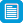# Search results

## Refine by topic

Main topic
Specific topic
Related topic
Listed under:  Mathematics  >  Statistics and probability  >  Probability  >  Expected frequency### Spinner

This is an interactive resource that investigates experimental and theoretical probability based on a spinner. The student can select the number of segments on the spinner and simulate spinning the spinner with a mouse click. The outcome of each trial is recorded in a table along with a comparison between the cumulative ...### reSolve: Scrabble Stats

This sequence of lessons invites students to collect data about letter frequency in a variety of text sources. They use their findings to critically evaluate letter point values in Scrabble, compare them to historical values, create their own themed Scrabble point values and to decipher an encoded excerpt of text. Each ...### Racing game with one die

This is an interactive game that investigates probability by simulating a two-car race, in which the movements of the cars are based on the roll of a die. Cars advance when certain numbers are rolled, and the student can experiment with probability by selecting which car moves forward for a given outcome of the roll of ...### Probability

This is a website designed for both teachers and students that addresses probability from the Australian Curriculum for year 7 students. It contains material on the language of probability, experiments and counting, and the probability of an event, and explains the mathematical use of the terms 'random' and 'randomly'. ...### Statistics games

This resource is a web page containing three dice games to explore chance. Each dice game has simple instructions to play the interactive strategy game. The games provide a useful way to investigate the chance of rolling a particular number after successive trials. This resource is one activity from the NRICH collection.### reSolve: Probability

This sequence of four lessons explores probability in real world situations including advertising, games and population sampling. Students calculate probabilities, represent probabilities as fractions, decimals and percentages, perform chance experiments with small and large sample sizes and graph their results, examine ...### reSolve: Probability - Rock Paper Scissors

In this sequence of two lessons, students determine their chances of winning the game 'rock paper scissors', then test their chances by playing against another player and in a simulated game. Students look at the psychological aspect of the game and recognise that there is a strategy to increase your chance of winning. ...